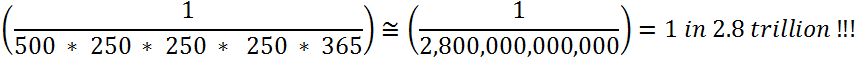# Myth and Math behind TNEA Random Number

The random number allocation in the TNEA counselling is a widely known process. But a few would have possibly dwelled into the Math behind this proces. And what’s more, all this requires just your +2 Math!

### Background

TNEA 2020 aggregate mark or cut-off mark is the sum of Physics mark obtained scaled to 50, Chemistry mark obtained scaled to 50 and Maths mark obtained scaled to 100. Thus the maximum aggregate or cut-off mark is 200. The candidates are ranked based on the aggregate mark or cut-off mark. The ranked candidates will be grouped into counselling sessions ordered by rank and in turn each counselling session will be ordered rank wise.

### Why

Many candidates will have same aggregate mark leading to tie, which should be broken to ensure unique ranking

### How

The following rules mentioned below are used to break the tie:

1. If two candidates have the same aggregate marks, the candidate with higher Maths mark will be ranked above
2. If the Maths Mark is same, then a higher Physics mark would break the tie
3. If the Maths and Physics mark are same, then the Chemistry mark would be eventually same. The fourth optional subject mark would break the tie.
4. If the fourth optional subject marks are also same then the candidate’s date of birth will be considered. Elder candidate gets the benefit of being ranked above.
5. If the date of birth is also same, then a 10-digit random number assigned to each candidate decides the ranking. A candidate with the highest random number will be ranked above the other.

### When

A few days before release of TNEA 2020 Rank list (7th September 2020, expected)

### Math behind Random Numbers

Let us plug in some numbers into this.

1. A student can secure cut-off marks in the range 70 – 200. 0.25 marks is the minimum increment. Hence there are 500+ possible ways an eligible student can secure cut-off marks
2. A student can secure Maths marks in the range 70 – 200 and minimum increment is 0.5 marks ( Hence 250+ ways
3. Similarly a student can secure marks in Chemistry and 4th optional subject in 250 X 250 ways
4. Chances that two candidates have the same Date of Birth is 1 in 365 (assuming both were born in same year)
5. Hence chances* that two students are actually allocated random numbers is* (1) assumed that the events are independent, though there should be overlaps.   (2) The marks is concentrated in the 150+ cut-off marks. However we assumed an uniform distribution.

### Reality

Students should not worry about random numbers. Chances are minimal that you will end up getting a lower random number.
The random number assignment process is totally transparent. The TNEA 2020 Random Number will be published public in the web on the specified date before the media persons. Along with the random number, the raw data captured from the coding sheet of the application form and more other information obtained during the application form process will be updated. After random number assignment, the rank list will be prepared and published in the website within few days.

Best Wishes,
TNEAhelp team